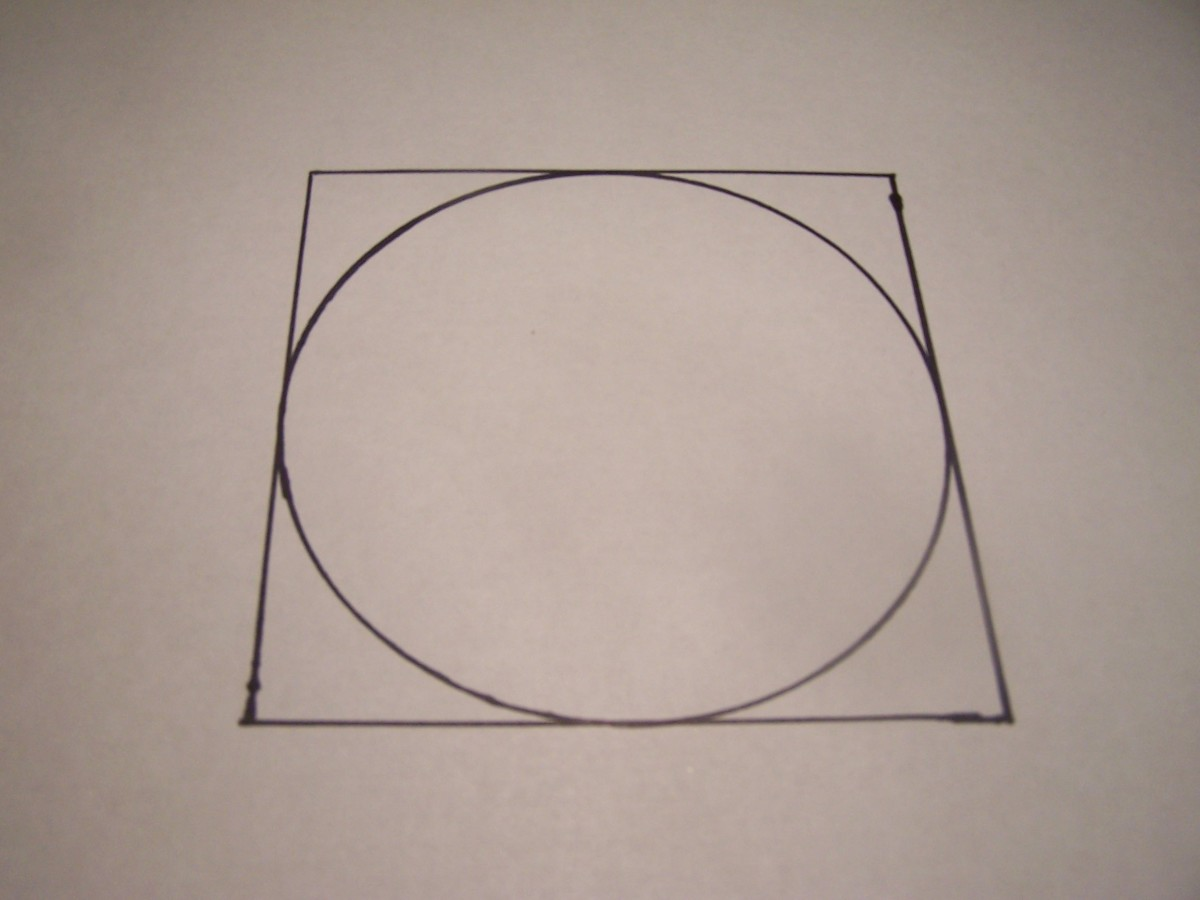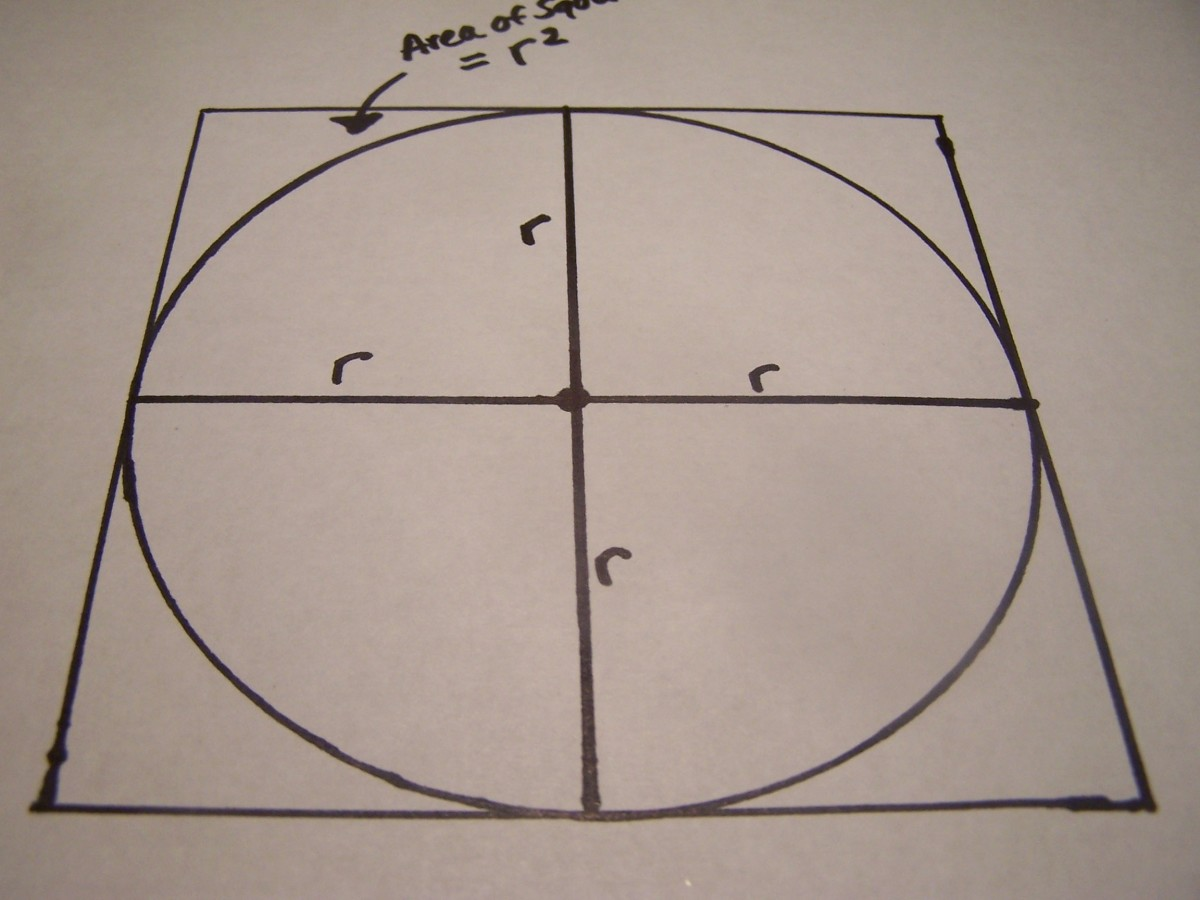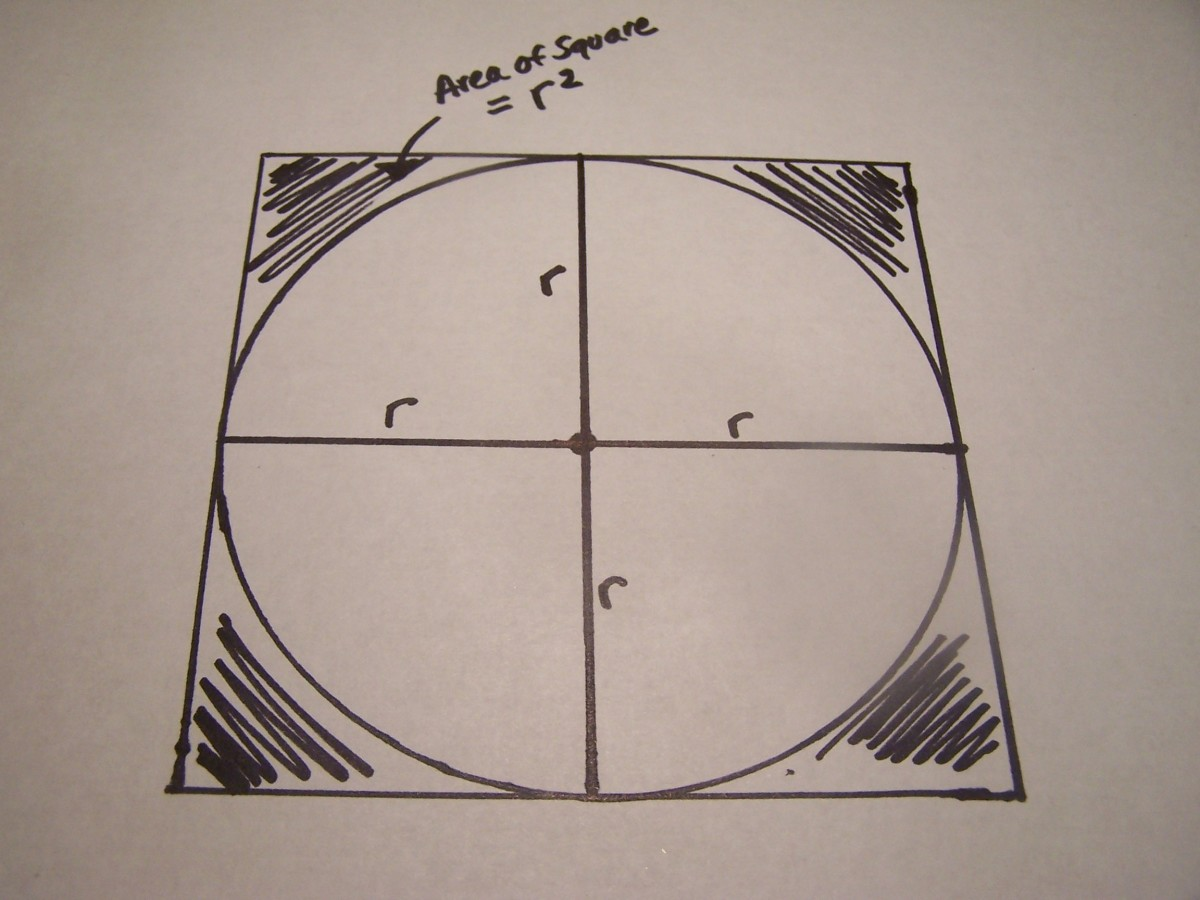# Math Made Easy! How to Find the Area of a Circle

## Geometry Tutorial:

Area of a Circle

When it comes to finding the area of geometric shapes, one problem that high school geometry students face is difficulty in remembering new terminology and formulas. This is especially true when it comes to the circle. New terms include: pi, radius, diameter, and circumference .

To make matters worse, the formulas for finding the area of a circle and the circumference of a circle look very similar and are often confused with one another.

Don't hurry out and find a geometry tutor just yet. This online geometry tutorial will:

• help you visualize the formula for finding the area of a circle,
• give you a Math Made Easy! tip on how to recognize the difference between the circle's area & circumference equations, and
• provide you with problems and solutions for finding the area of a circle.

How to find the:

## Area of Circle Formula

A = π r2

Geometry Circle Terms to Know:

• A: Area
• π: 3.14 (pronounced pi)
• r: radius (the distance from the center of a circle to a point on its edge)
• d: diameter (the distance across a circle going through its center; it is twice the radius)
• C: Circumference (the distance around a circle, in other words, the perimeter of the circle)

## Understanding Where a Formula Comes From Makes it Easier to Remember It!Notice the area of the circle is slightly smaller than the area of the large square in which it fits perfectly inside.ktrapp
Scroll to ContinueDraw two more radii "r" and notice that there are now 4 small squares. Since the area of one small square is 1-r-squared, the total area of the 4 small squares equals 4-r-squared.ktrappTherefore, the area of the large square is 4-r-squared. The circle's area is slightly smaller and is (3.14)-r-squared or (pi)-r-squared.ktrapp

## How the Equation for the Area of a Circle is Derived

Have you ever wondered why the equation of a circle is A = πr2 ?

• Notice the circle which fits perfectly inside the big square. The radius of the circle is r.
• Let's draw a second radius. Notice that a small square is now formed. The lengths of each side of the small square equal r.
• The area of the small square is r2 since the equation for the area of a square is length times width. In the case of our small square the area is r times r, which simplifies to r2. For a moment think of the area of the small square as 1r2.
• Let's draw some more radii (plural of radius). Now we have 4 small squares and each small square has an area of 1r2. The total area of the 4 small squares, therefore, equals 4r2.
• Since the 4 small squares are the same size as the 1 large square, the area of the large square is also equal to 4r2.
• The circle is slightly smaller than the large square so the area of the circle is less than the area of the large square. We know that the area of the square is 4r2 and as it turns out the area of the circle is about 3r2.
• Mathematicians know that the exact area of a circle is actually closer to 3.14r2 and since π = 3.14 the formula for finding the area of a circle is written as πr2.

How to remember the difference between a circle's area and circumference formulas.

• Area of Circle = πr2
• Circumference of Circle = 2πr

Yikes! Both of those equations look very similar to each other. But don't worry.

There are two easy ways to remember the difference between the area of a circle equation and the circumference of a circle equation:

1. Area is always measured in squared terms. For example a 10 foot X 10 foot room equals 100 square feet. The area of a rectangle with sides of 5 units and 10 units equals 50 square units. You can therefore remember that the circle equation for area is the one that is squared.
2. Visualize a circle that fits perfectly inside of a square. Remember that the area of the square is 4r2 and the area of the circle is smaller, about 3r2.

## Geometry Help Online: Area of Circle

Check out three common geometry homework problems for finding the area of a circle below. Solutions and answers are provided.

## Math Made Easy! Quiz - Area of Circle

For each question, choose the best answer. The answer key is below.

1. What is the area of a circle with a radius of 3 cm?
• 88.74 cm. squared
• 28.26 cm. squared
• 18.84 cm. squared
2. What is the area of a circle with a radius of 8 ft?
• 200.96 square ft.
• 50.24 square ft.
• 157.75 square ft.

1. 28.26 cm. squared
2. 200.96 square ft.

## #1 Find the Area of a Circle Given the Radius

Problem: Find the area of a circle with a radius of 5 units.

Solution: Plug in 5 for r in the formula A = πr2 and solve.

• A = π52
• A = 25π (Follow the order of operations and square 5 before multiplying it by pi.)
• A = (25)(3.14)
• A = 78.5

Answer: The area of a circle with a radius of 5 units is 78.5 square units.

## #2 Find the Area of a Circle Given the Diameter

Problem: A circle has a diameter of 4 meters. What is the area of the circle?

Solution: The diameter is the measure across the circle through its center. The radius is the measure from the center of the circle to its edge. Therefore, the radius is 1/2 the diameter. Since the diameter of the circle is 4 meters, its radius is 2 meters. Plug in 2 for r in the area of a circle formula and solve.

• A = π22
• A = 4π
• A = (4)(3.14)
• A = 12.56

Answer: The area of a circle with a diameter of 4 meters is 12.56 meters squared.

## #3 Find the Area of a Circle Given the Circumference

Problem: A circle has a circumference (perimeter) of 100 meters. What is the circle's area?

Solution: When figuring out the area of a circle you need to find the radius to plug into the area formula. In this example we only know the circumference. Let's plug in the known circumference (100) into the circumference of a circle formula and solve for r:

• 100 = 2πr
• 100 = (2)(3.14)r
• 100 = 6.28r
• r = 15.92 (divide both sides by 6.28)

Now, that we know the radius equals 15.92, let's plug r into the area of a circle formula and solve:

• A = π(15.92)2
• A = 253.45π
• A = (253.45)(3.14)
• A = 795.83

Answer: The area of a circle with a circumference of 100 meters is about 796 square meters.

## Do you need more geometry help online?

If you have other types of problems you need help with related to the area of a circle please ask in the comment section below. I'll be glad to help out and may even include your area of a circle problem in the problem/solution section above.

Kristin Trapp (author) from Illinois on April 28, 2012:

mrbrianmclogan - I've never forgotten the formula for the area of a circle once I could visualize it. I'm glad the Hub provided enough detail.

mrbrianmclogan from Jacksonville on April 28, 2012:

Great Hub, thank you for sharing. You provided great detail

Kristin Trapp (author) from Illinois on February 23, 2012:

math-worksheets - That's very generous of you to say that this is one of the best "area of a circle" tutorials that you've seen. I appreciate it and I hope it helps students.

math-worksheets from California on February 23, 2012:

This is one of the best tutorials for finding the area of circle! Really good tutorial! I don't know why I haven't find it before to end up my misery.

Kristin Trapp (author) from Illinois on October 27, 2011:

Thank you Deborah. Hopefully, high school kids needing Geometry help will find their way to this tutorial if need be. Thanks for commenting.

Deborah Neyens from Iowa on October 27, 2011:

I hope never to need this knowledge, but had to check out your hub anyway. What a great idea to do a series of geometry tutorials! : )

Kristin Trapp (author) from Illinois on October 24, 2011:

Thanks homesteadbound. I really liked algebra too. I guess what I always liked was that you knew if you got the correct answer or not because you could simply plug the answer back into the equation to see if it all was true. The exactness of algebra and math was always appealing to me.

Cindy Murdoch from Texas on October 24, 2011:

This is a great tutorial. I must admit however that math was not one of my favorite subjects. I kind of liked algebra however.